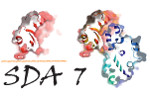SDA (SDA flex)  7.2 Simulation of Diffusional Association
mod_geometry::geometry Type Reference

geometry is a base (common base ) class More...

Collaboration diagram for mod_geometry::geometry:

## Public Attributes

integer type
type of the contained geometry : box or sphere defined by enumeration More...

type(sphere), pointer pshere
pointer to a sphere geometry More...

type(box), pointer pbox
pointer to a box geometry More...

real(kind=4) min_height
If using spherical geometry with a surface, at what height should the spherical cap b surface start above the surface. More...

logical half_sphere
Apply half_sphere even when not using a surface. More...

logical record_startpos
recording of start and end positions of simulations More...

logical escape
properties of the geometry object If the solutes can escpape, if there is pbc, if there is a surface More...

logical pbc

logical surface

real(kind=4) start_position
starting and escaping position. redondant wit b and c surface More...

real(kind=4) stop_position

## Detailed Description

geometry is a base (common base ) class

## ◆ escape

 logical mod_geometry::geometry::escape

properties of the geometry object If the solutes can escpape, if there is pbc, if there is a surface

## ◆ half_sphere

 logical mod_geometry::geometry::half_sphere

Apply half_sphere even when not using a surface.

## ◆ min_height

 real ( kind=4 ) mod_geometry::geometry::min_height

If using spherical geometry with a surface, at what height should the spherical cap b surface start above the surface.

## ◆ pbc

 logical mod_geometry::geometry::pbc

## ◆ pbox

 type( box ), pointer mod_geometry::geometry::pbox

pointer to a box geometry

## ◆ pshere

 type( sphere ), pointer mod_geometry::geometry::pshere

pointer to a sphere geometry

## ◆ record_startpos

 logical mod_geometry::geometry::record_startpos

recording of start and end positions of simulations

## ◆ start_position

 real ( kind=4 ) mod_geometry::geometry::start_position

starting and escaping position. redondant wit b and c surface

## ◆ stop_position

 real ( kind=4 ) mod_geometry::geometry::stop_position

## ◆ surface

 logical mod_geometry::geometry::surface

## ◆ type

 integer mod_geometry::geometry::type

type of the contained geometry : box or sphere defined by enumeration

The documentation for this type was generated from the following file:
Imprint/Privacy